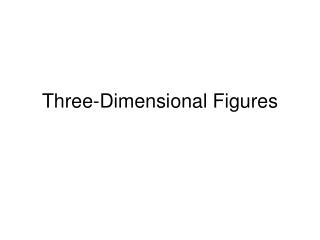DownloadDownload PresentationThree-Dimensional Figures

# Three-Dimensional Figures

Download Presentation## Three-Dimensional Figures

- - - - - - - - - - - - - - - - - - - - - - - - - - - E N D - - - - - - - - - - - - - - - - - - - - - - - - - - -
##### Presentation Transcript

1. Three-Dimensional Figures

2. Vocabulary • Two-dimensional figures (plane figures) – triangles, quadrilaterals, and circles. They lie in one plane

3. Vocabulary • Three-dimensional figures – figures that have length, width, and depth • Cone • Cylinder • Prism • Pyramid • Sphere

4. How are 3-D figures created?

5. Two-dimensional figures can be transformed through space to create three-dimensional figures. One way is to rotate a two-dimensional figure about a line. This line is sometimes called an axis of symmetry.

6. Space Figures • Translate a rectangle through space – It creates a rectangular prism!

7. Space Figures • Translate a triangle through space – It makes a triangular prism!

8. Space Figures • Translate a circle through space – It makes a cylinder!

9. Space Figures • Rotate a rectangle around an axis of symmetry – it also makes a cylinder!

10. Space Figures • Twirl (rotate) a triangle around one of its sides – It makes a cone!

11. Space Figures • Twirl (rotate) a circle around its diameter – It makes a sphere!

12. Let’s try some examples! Example 1

13. Solution

14. Example 2

15. Solution

16. Example 3 A square measures 1 inch on each side. Suppose a copy of the square is translated 2 inches directly above the plane in which it lies to form a second square. The two squares are then connected with four line segments. Describe the three-dimensional figure that is formed.

17. Solution

18. Example 4

19. Solution

20. Example 5

22. 1. What is the figure show below called? • Cone • Cylinder • Cube • Rectangular prism

23. 2. The base of a cylinder is shaped like a ______. • Rectangle • Square • Triangle • circle

24. 3. What three-dimensional figure will be formed if the right triangle shown is rotated about the line shown? • Cone • Cylinder • Cube • sphere

25. 4. What three-dimensional figure will be formed if the rectangle shown is rotated about the line shown? • Cone • Cylinder • Cube • sphere

26. 5. A rectangle is translated 3 inches directly above the plane in which it lies. The vertices of the two rectangles are then connected with four line segments. What three-dimensional figure is formed? • Cone • Cube • Cylinder • Rectangular prism

27. 6. What three-dimensional figure will be formed if rectangle ABCD is rotated about AB? • Cone • Cube • Cylinder • prism

28. Ticket-out-the-door • What 3D figure is created when you…. • Translate a rectangle • Translate a circle • Rotate a rectangle • Translate a triangle • Rotate a triangle • Rotate a circle • Translate a square

29. Cross-Sections EQ: How can I describe the cross-sections of solid figures?

30. Vocabulary • Cross-section – a view of the inside of a 3D figure after it is sliced • Polyhedron – a 3D figure (a solid with flat faces) http://www.learner.org/channel/courses/learningmath/geometry/session9/part_c/index.html

31. Example 1 Describe three different cross-sections you can find for the rectangular prism below

32. Solution

33. Example 2 The cylinder below sits on a horizontal base. Draw and describe the cross-section formed when the cylinder is cut by a plane that is tilted away from its base.

34. Solution • The cross-section is shaped like an oval. In mathematics, this shape is called an ellipse.

35. Example 3 • The cone below sits on a horizontal base. Draw and describe the cross-sections formed when the cone is cut by a vertical plane through its vertex.

36. Solution • The cross-section is shaped like an isosceles triangle. The base of the triangle is on the base of the cone.

37. Example 4 • The pyramid below has a square horizontal base. Draw and describe the cross-sections formed when the cone is cut by a vertical plane that does not pass through the vertex at its top.

38. Solution • The cross-section will be shaped like a quadrilateral. This figure is called an isosceles trapezoid.

39. Activity